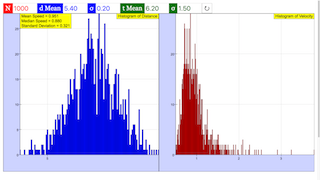Intro Page

Monte Carlo error propagation

Developed by Andy Runquist

This set of exercises guides the student in exploring how to use a computer algebra system to determine the propagated error of a calculated parameter based on measured quantities with known uncertainties. This approach is based on the Monte Carlo approach.

As is detailed very thoroughly here, there are many methods for doing error propagation. Probably the most common is the calculus approach which assumes that not only do all variables follow a normal distribution, but that any calculation does so as well. Note how the examples described here don’t obey that latter issue. The link above carefully describes how the Monte Carlo method is the most accurate way of doing error propagation. From a numeric perspective, it’s also the easiest (not counting the simple crank-three-times). Certainly using a Computer Algebra System allows for coding up the calculus approach, but if the Monte Carlo approach is more accurate, why not do it and skip having to use a Computer Algebra System?

 Subject Area Mathematical/Numerical Methods First Year Mathematica and Python Students will be able to: Generate normally distributed random numbers (Exercise 1) Plot histograms. Calculate mean, median, and standard deviation for a distribution. Generate a new distribution from previously generated random numbers. (Exercise 3) Compare the analytical (calculus) approach to the Monte Carlo approach (Exercises 2 and 3) 30 min
Exercise
1. Produce a large set of numbers that obey a normal distribution with a mean of 5.4 and a standard deviation of 0.2. Many computer programming languages have a weighted random number built in. You can also build your own using the Box Muller transformation that takes two uniformly distributed random numbers between 0 and 1 and returns two normally distributed random numbers with a mean of zero and a standard deviation of 1. These can then be transformed to match the requested mean and standard deviation. Plot a histogram of the large data set, confirming that the peak and width are what you expected.
2. Determine the histogram for the speed example above by using the calculus error propagation approach.

a) Assuming that all values are distributed according to a normal distribution, the calculus approach is given by

$\begin{array}{}\text{(2)}& {\sigma }_{f}=\sqrt{{\left(\frac{\mathrm{\partial }f}{\mathrm{\partial }x}{\sigma }_{x}\right)}^{2}+{\left(\frac{\mathrm{\partial }f}{\mathrm{\partial }y}{\sigma }_{y}\right)}^{2}}\end{array}$
Show that in the case of the speed calculation, this becomes :
$\begin{array}{}\text{(3)}& {\sigma }_{v}=\frac{x}{t}\sqrt{{\left(\frac{{\sigma }_{x}}{x}\right)}^{2}+{\left(\frac{{\sigma }_{y}}{y}\right)}^{2}}\end{array}$

b) Using Eq. 2, determine the error for the speed example from Exercise 1. 3. Now use the Monte Carlo method. Generate several hundreds or thousands of values for both position and time according to their respective distributions. Calculate the speed that corresponds to each of these values, and plot a histogram of speeds. Compare with the results of exercise 2.

3. Produce several (hundreds or thousands) of both position and time estimates according to their respective distributions and calculate their associated speeds. Then plot the histogram of those speeds and compare with (2).

4. Determine the mean, median, and standard deviation of the histrogram for (3) and compare with 2.
5. Extend the Monte Carlo approach to lab data of your own.
montecarlo.py

#!/usr/bin/env python

'''

montecarlo.py

Eric Ayars

June 2016

Python solutions to PICUP "Monte Carlo error propagation"

exercise set.

'''

from pylab import *

import random

#########################

# Exercise 1

#########################

# Generate the gaussian distribution

N = 1000 # How many to generate

mu = 5.4 # mean

sigma = 0.2 # sigma

dist = array([random.gauss(mu, sigma) for j in range(N)])

# Plot a histogram of the distribution

hist(dist, 30)

show()

#########################

# Exercise 3

#########################

# characteristits of d and t

avg_d = 5.4

sigma_d = 0.2

avg_t = 6.2

sigma_t = 1.5

N = 1000 # How many to generate

# Generate distribution of distances

d = array([random.gauss(avg_d, sigma_d) for j in range(N)])

t = array([random.gauss(avg_t, sigma_t) for j in range(N)])

# Since d and t are numpy arrays, we can just divide, the

# resulting index-wise multiplication will generate another

# numpy array of speeds.

v = d/t

# plot histogram of velocity

hist(v, 30)

show()

#########################

# Exercise 4

#########################

# report average and sigma

print('mean speed = %0.3f' % mean(v))

print('median speed = %0.3f' % median(v))

print('standard deviation = %0.3f' % std(v))

### Translations

Code Language Translator Run### Software Requirements

SoftwareRequirements

 Android iOS Windows MacOS with best with Chrome Chrome Chrome Chrome support full-screen? Yes. Chrome/Opera No. Firefox/ Samsung Internet Not yet Yes Yes cannot work on some mobile browser that don't understand JavaScript such as..... cannot work on Internet Explorer 9 and below

### CreditsFremont Teng; Loo Kang Wee; based on codes by Andy Runquist

[text]